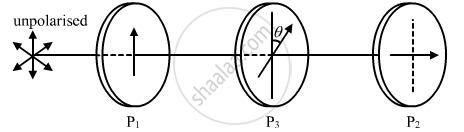Share

# Find an Expression for Intensity of Transmitted Light When a Polaroid Sheet is Rotated Between Two Crossed Polaroids. - CBSE (Science) Class 12 - Physics

#### Question

Find an expression for intensity of transmitted light when a polaroid sheet is rotated between two crossed polaroids. In which position of the polaroid sheet will the transmitted intensity be maximum?

#### SolutionLet us consider two crossed polarisers P1 and P2, with a polaroid sheet P3 placed between them.

Let I0 be the intensity of polarised light after passing through the first polariser P1. If θ is the angle between the axes of P1 and P3, then the intensity of the polarised light after passing through P3 will be I=I0cos2θ.

As P1 and P2 are crossed, the angle between the axes of P1 and P2 = 90°.

∴ Angle between the axes of P2 and  P3 = (90°θ)

The intensity of light emerging from P2 will be given by

I=[I0cos2θ]cos2(90°θ)

I=[I0cos2θ]sin2θ

=>I=I_0/4(4cos^2thetasin^2theta)

=>I=I_0/4(2sinthetacostheta^2)

=>I=I_0/4sin^2theta

The intensity of polarised light transmitted from P2 will be maximum when

sin2θ=maximum=1

sin2θ=sin90°

2θ=90°

θ=45°

Also, the maximum transmitted intensity will be given by

I=I_0/4

Is there an error in this question or solution?

#### Video TutorialsVIEW ALL 

Solution Find an Expression for Intensity of Transmitted Light When a Polaroid Sheet is Rotated Between Two Crossed Polaroids. Concept: Polarisation.
S